# 为什么要学习闭包和装饰器

## 闭包

### 什么是闭包

``````def test1():
num = 1
def test2():
print(num)

return test2

result = test1()
result()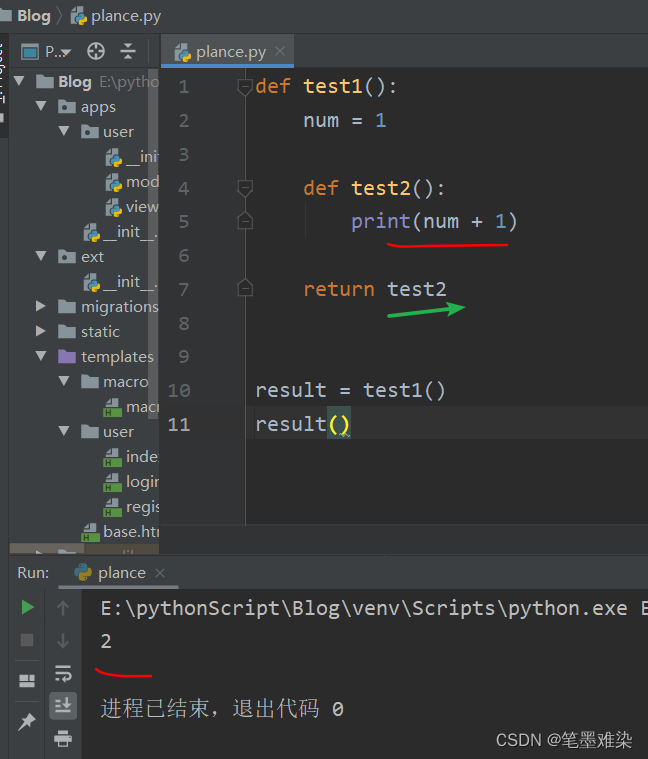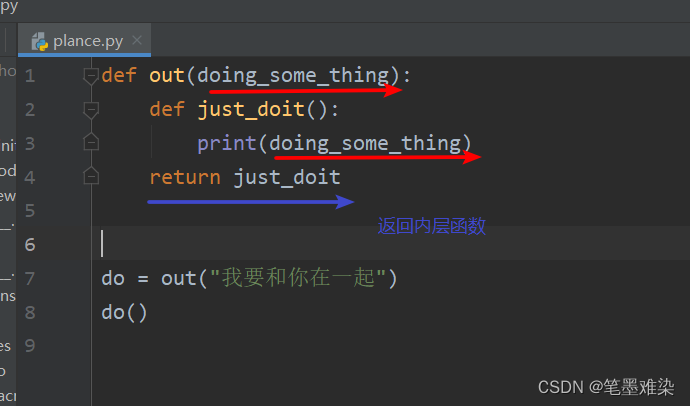### 闭包的注意事项(一)如果要对外层的变量进行修改, 就需要使用 nonlocal var 进行声明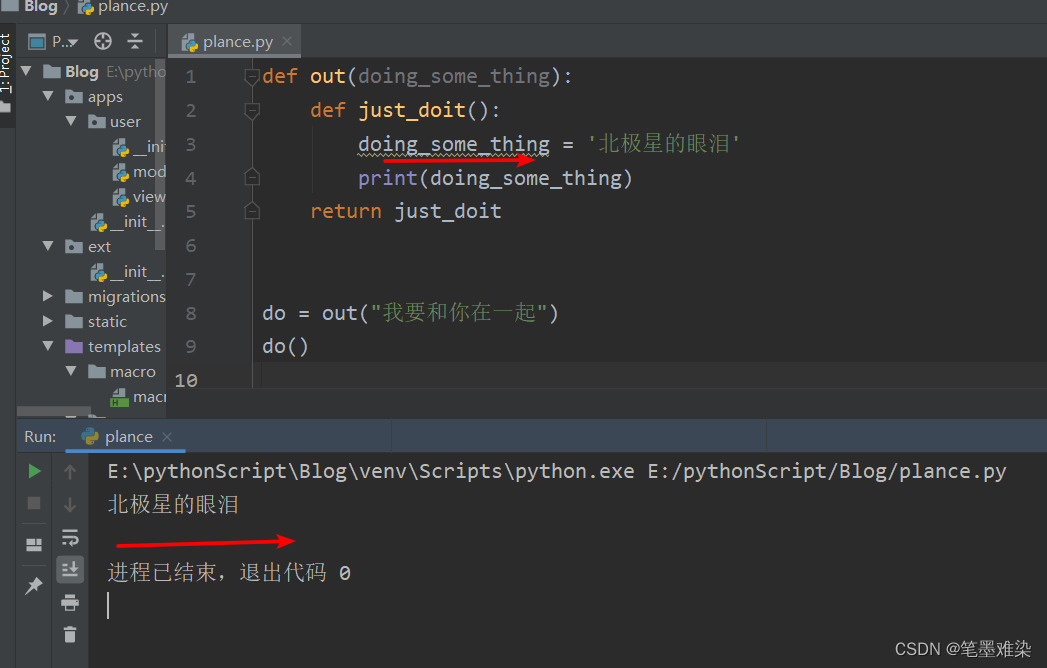``````def test1():
a = 10
def test2():
nonlocal a
a = 11
print("修改外层变量")
print(a)

# 未修改之前
print("未修改之前" + str(a))
# 修改外层变量
test2()
# 修改之后
print("修改外层变量后" + str(a))
return test2

test1()``````

### 闭包的注意事项(二)当闭包内,引用了一个, 后期会发生变化的变量时,一定要注意

``````def test(a):
a = 1
def test2():
print(a)
a = 2
return test2
newFunc = test(5)
newFunc()``````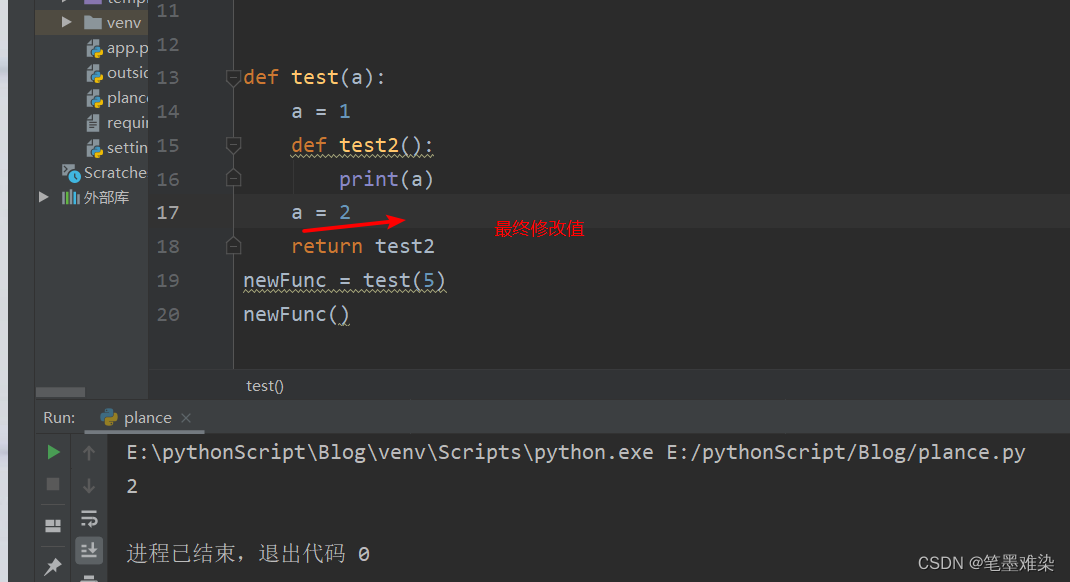### 闭包的深入用法

``````def test():
funcs = []
for i in range(1, 4):
def test2(num):
num = 1 + i
def inner():
print(num)
return inner
funcs.append(test2(i))
return funcs
newFuncs = test()
print(newFuncs)
newFuncs()
newFuncs()
newFuncs()``````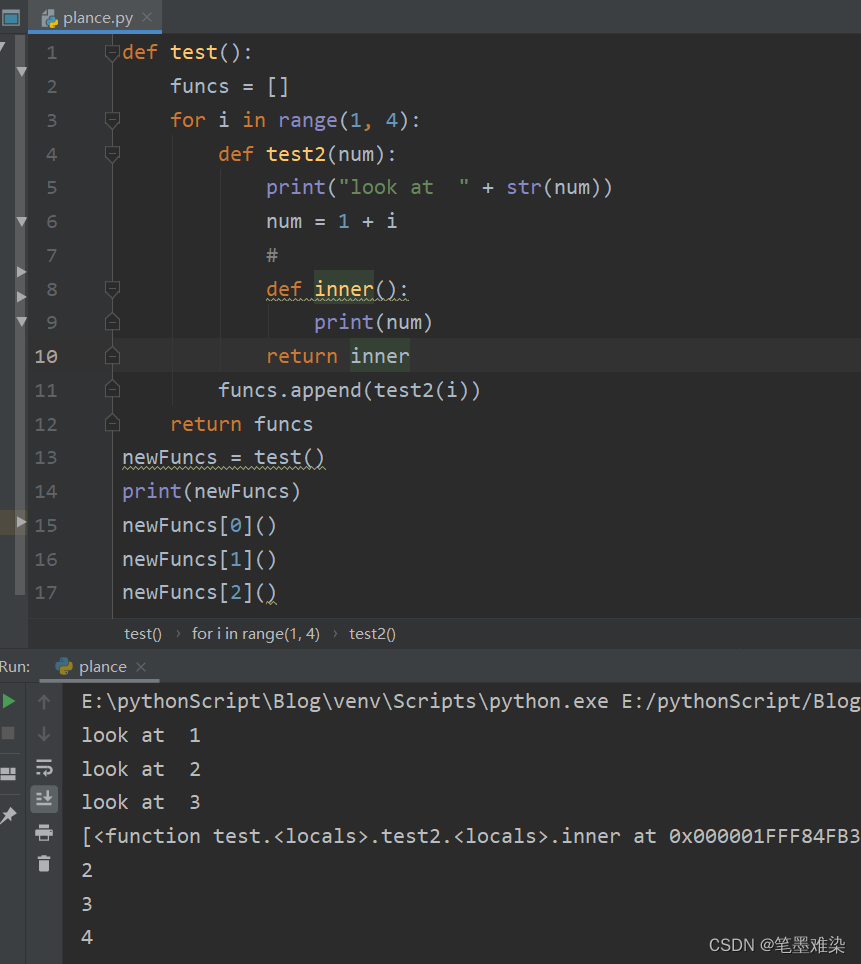## 装饰器

### 装饰器的作用:

``````def fss():
print("发说说")
def ftp():
print("发图片")``````

### 引出装饰器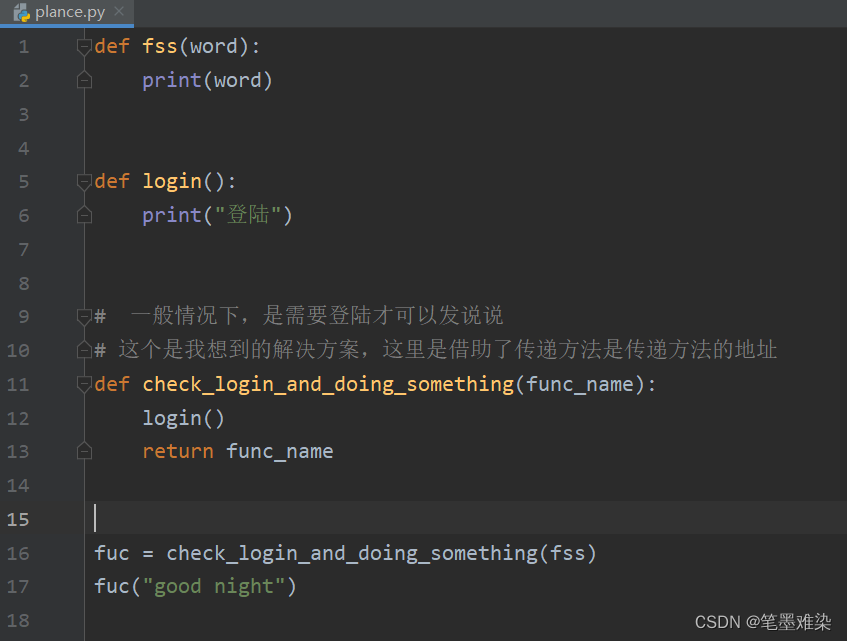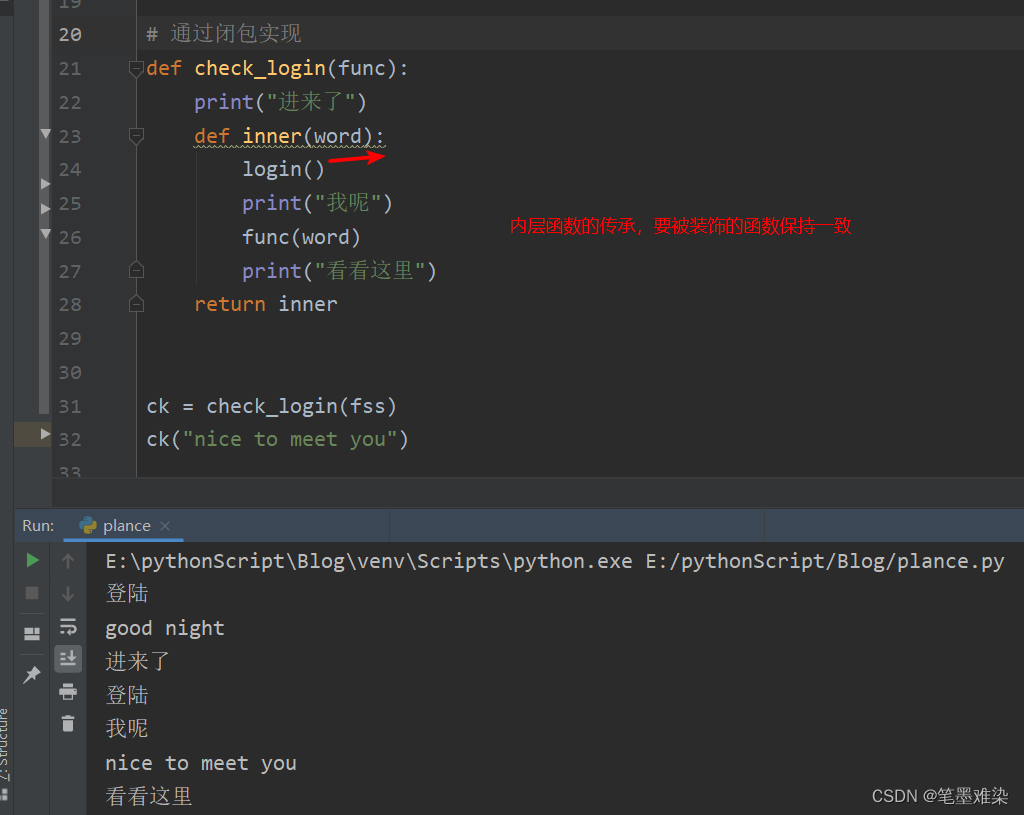### 装饰器的语法糖

``````def check_login(func):
def inner():
print("测试登陆")
func()
return inner
def say_word():
print("发说说")
``````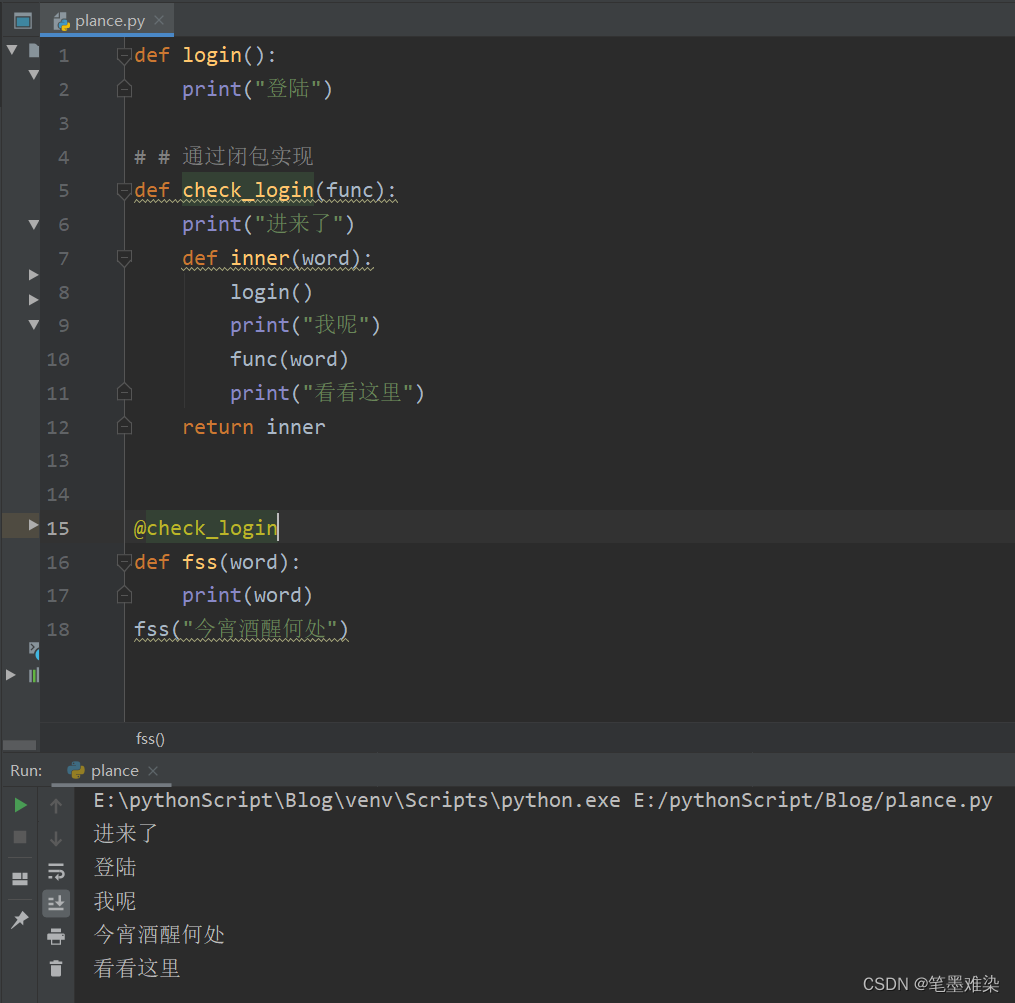### 带参数的装饰器

``````def get_zsq(char):
def zsq(func):
def inner():
print(char * 10)
func()
return inner
return zsq

@get_zsq("\$") #语法糖, 相当于 fn_num = zsq(fn_num), 而且装饰器语法糖不支持多个传参, 会报错
def fn_num():
print("123")

fn_num()

def where_are_you_from(country):
def where_city(func):
def inner(city, number):
print("i am from " + country + "and my home in" + city + "and " + str(number) + " years")
# 这一步不要少了，不然被装饰的函数就不会执行了， 而且参数一致
func(city, number)
return inner
return where_city

@where_are_you_from("china")
def get_views(city, number):

get_views("shenzhen", '3')

``````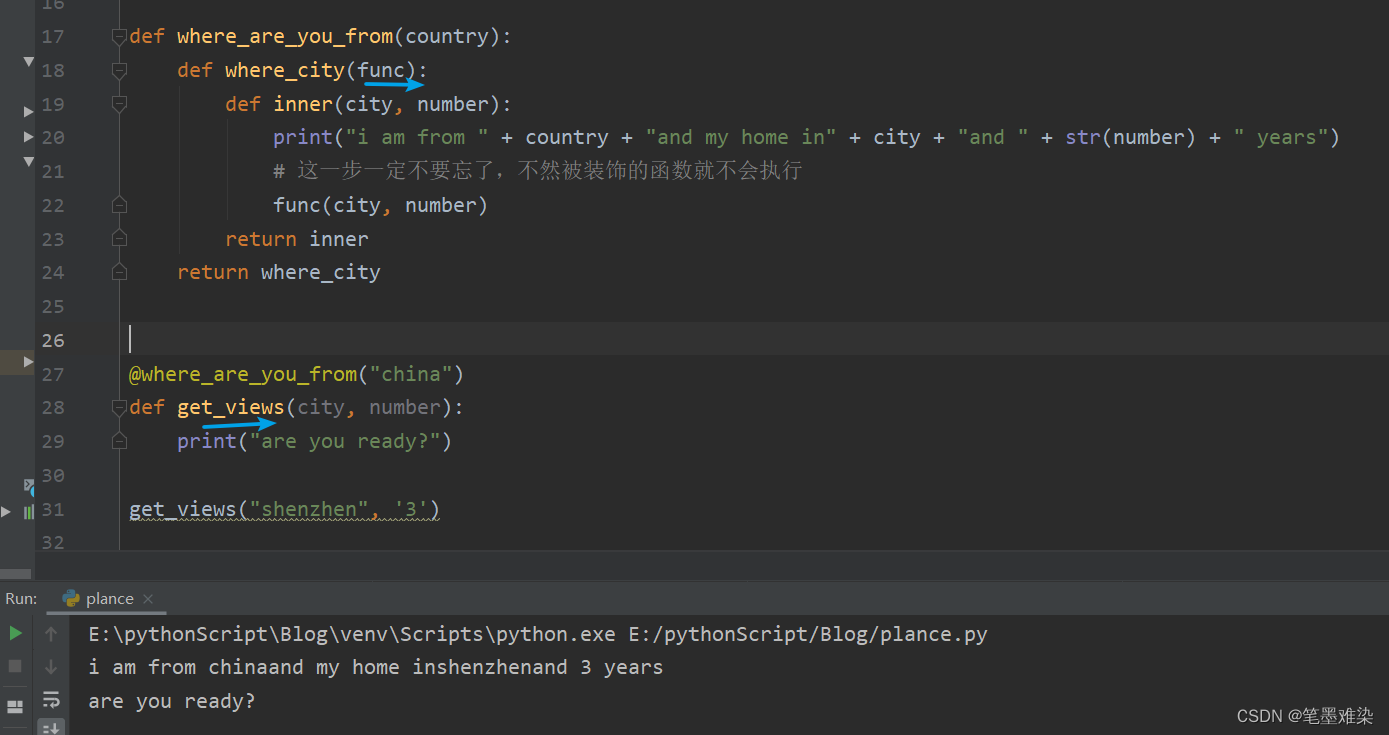### 装饰器的叠加和执行顺序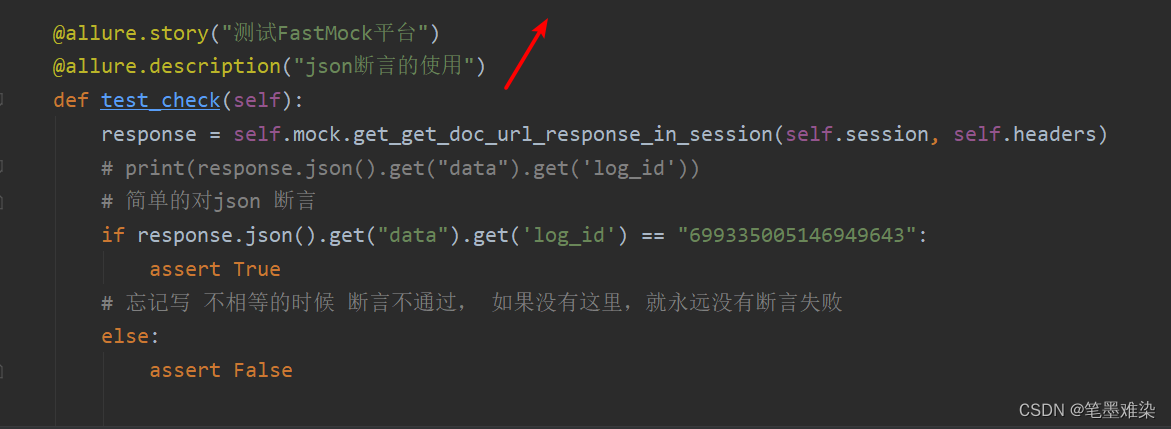### 被装饰的函数带参数，而且参数个数不固定怎么办

``````def zsq(func):
def inner(*args, **kwargs):
print("_" * 30)
print(args, kwargs)
func(*args, **kwargs)
return inner

# 复习 *args
@zsq
def pnum(num, num2, num3):
print(num, num2, num3)
# 复习 **kwargs
@zsq
def go_home(*args, **kwargs):
print("调用完了")

pnum(15, 18, 20)
go_home("深圳", "北京", country="china", provins='广东')

``````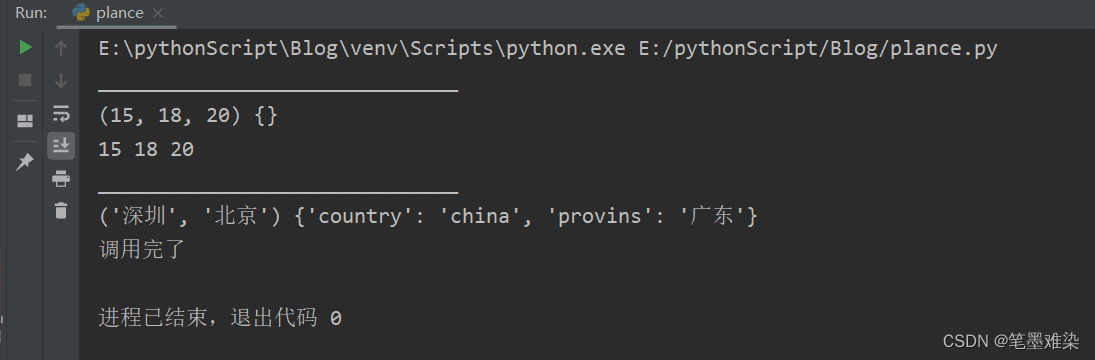### 被装饰的函数有返回值怎么办？

``````def judge_login(func):
def inner(*args):
result = func(*args)
# 返回
return result
return inner

def fss(word):
print(word)
# 返回None
return

def fsp(word, news):
print(word, news)
# 有返回值
return "nothing is"
print("====")
result1 = fss("你好")
print(result1)
result2 = fsp("哈哈","晚上")
print(result2)

``````

### 跨模块引用装饰器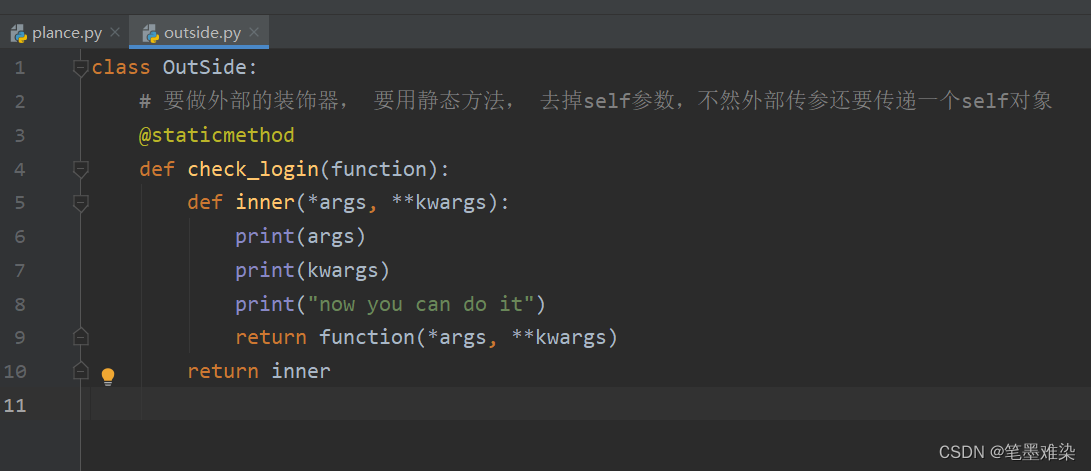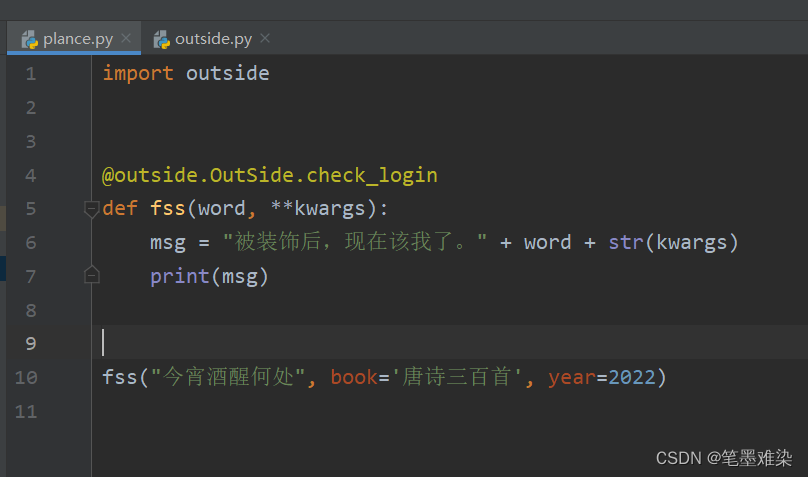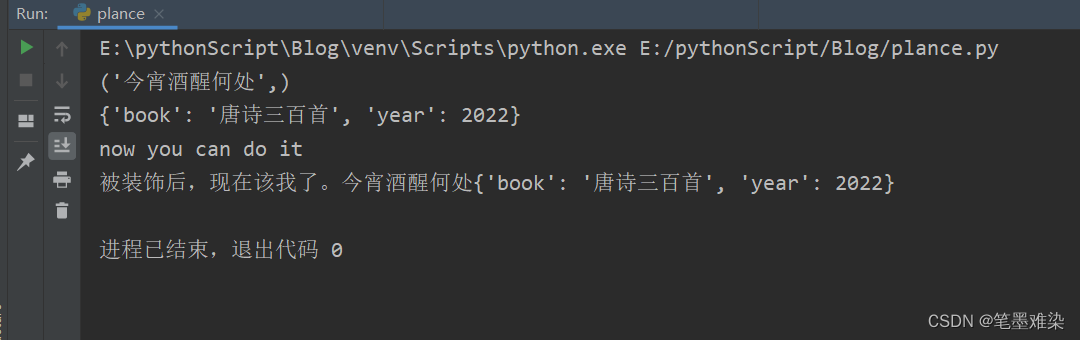目录